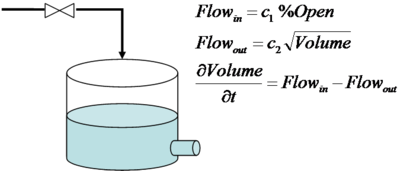## Tank Model

The same dynamic tank model is written in the following 4 modeling languages for a direct comparison between the syntax for the solution of differential and algebraic equations.

• APMonitor
• MATLAB
• gProms
• ModelicaParameters percent_open a1 = 0.25 ! m^3/sec a2 = 0.14 ! m^1.5/sec Variables inlet_flow ! m^3/sec outlet_flow ! m^3/sec volume ! m^3 Equations inlet_flow = a1 * percent_open outlet_flow = a2 * SQRT(volume) $volume = inlet_flow - outlet_flow 200 < volume < 5000 ### Tank Model in MATLAB  function xdot = tank(t,x) global u % Input (1): % Inlet Valve State (% Open) percent_open = u; % State (1): % Volume in the Tank (m^3) volume = x; % Parameters (2): % Inflow Constant (m^3/sec) c1 = 0.25; % Outflow Constant (m^1.5/sec) c2 = 0.14; % Intermediate variables inlet_flow = (c1 * percent_open); outlet_flow = (c2 * volume^0.5); % Compute xdot (dx/dt) xdot(1,1) = inlet_flow - outlet_flow; % adjust xdot to remain within constraints low_volume = 200; low_open = c_outflow * low_volume^0.5)/c_inflow; if (volume < low_volume) & (percent_open < low_open), xdot(1,1) = 0; end high_volume = 5000; high_open = c_outflow * high_volume^0.5)/c_inflow; if (volume > high_volume) & (percent_open > high_open), xdot(1,1) = 0; end  ### Tank Model in gProms  MODEL Tank  DECLARE  TYPE Vol = 500.0 : 200.0 : 5000 UNIT = "m^3" END PARAMETER # parameters can be specified at run-time # with Tank.c1 := 0.25; # Tank.c2 := 0.14; c1 AS REAL c2 AS REAL VARIABLE # Volume in the Tank (m^3) volume AS Vol # Inlet flow (m^3/sec) inlet_flow AS REAL # Outlet flow (m3/sec) outlet_flow AS REAL # Inlet Valve State (% Open) percent_open AS REAL EQUATION # Inlet flow is a linear function of valve position inlet_flow = c1 * percent_open ; # Square root pressure drop flow relation outlet_flow = c2 * SQRT ( volume ) ; # Mass balance (assuming constant density)$volume = inlet_flow - outlet_flow ;

END # Model Tank


### Tank Model in Modelica

 model Tank
parameter Real c1=0.25 "Inflow Constant";
parameter Real c2=0.14 "Outflow Constant";
Real percent_open "Percent Open";
Real inlet_flow "Inlet Flow";
Real outlet_flow "Outlet Flow";
Real volume "Tank Volume";
equation
inlet_flow = c1 * percent_open "Inlet flow";
outlet_flow = c2 * volume^0.5 "Outlet flow";
der(volume) = inlet_flow - outlet_flow "Mass balance";
end Tank;